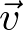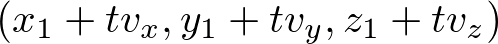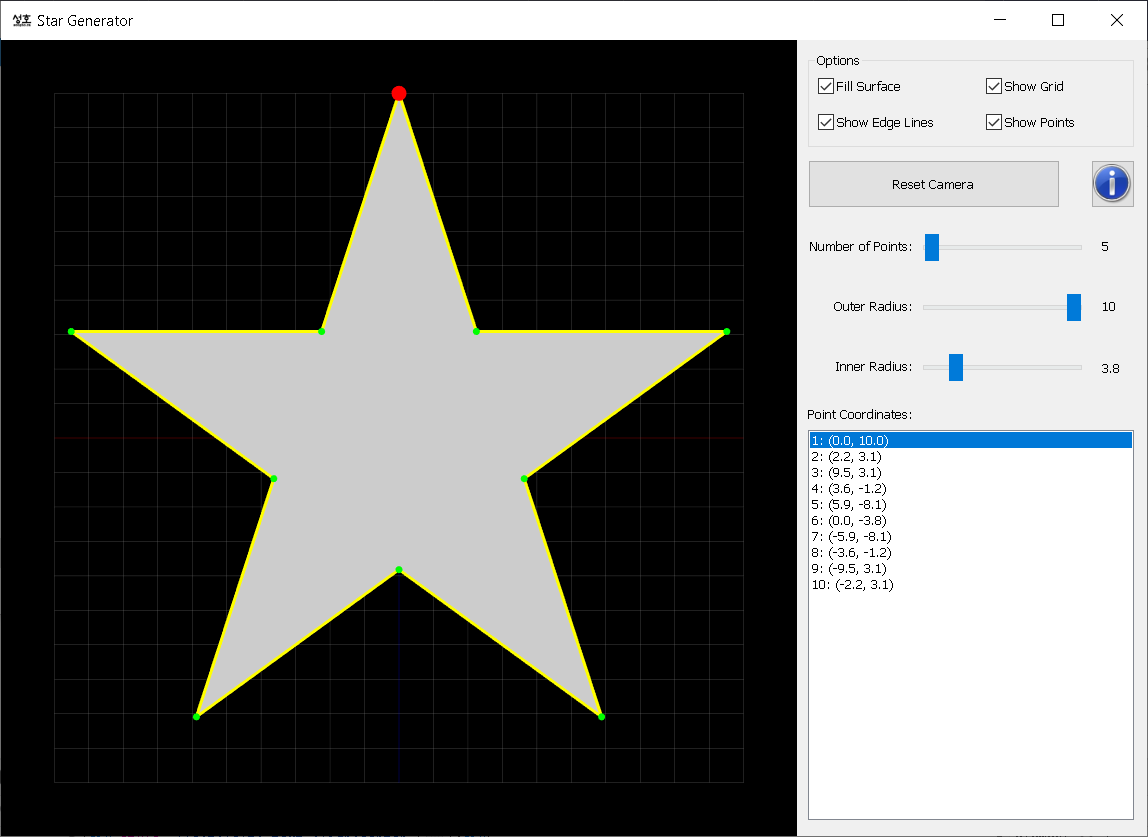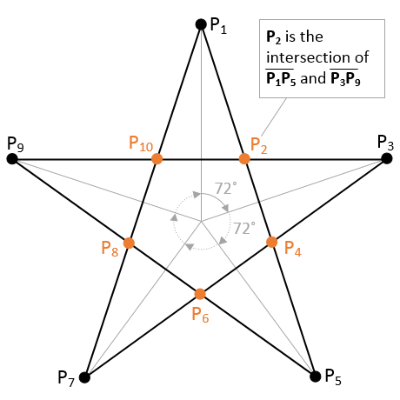# Line Equation

Related Topics: Plane EquationGraph of a line

The equation of a line is defined with 2 known points. This page describes a line equation using parametric form; a pointon the line and the direction vectorof the line.

The direction vector can be computed by subtracting 2 known points;Therefore, the parametric form of the line equation is;Or, each element (x, y, z) of the line can be written by the parameter t;Notice that it is same as linear interpolation formula.

Also note that 2D line equation is frequently represented with slope-intercept formor standard form. However these forms are not suitable for computer algorithms; the slope of a vertical line is undefined (±∞), and these forms cannot be used in 3D space.

### Intersection of 2 LinesIntersection of 2 Lines

The intersection point of 2 lines is solving the linear system of 2 lines using vector algebra.First, solve the linear system, Line1 = Line2 for t. Then substitute t into Line1 equation to find the intersection point (x, y, z).

(Reference: Intersection of two lines in three-space, Ronald Goldman, Graphics Gems, page 304, 1990)If two lines are parallel, the cross product of 2 direction vectors of the lines becomes zero. You can determine if two lines are intersect or not with;Here is C++ code to find the intersection point of 2 lines. Please see the details in Line.cpp.

``````
// dependency: Vector3, Line
struct Vector3
{
float x;
float y;
float z;
};

class Line
{
Vector3 direction;                 // (Vx, Vy, Vz)
Vector3 point;                     // (Px, Py, Pz)
};

Vector3 intersect(Line& line1, Line& line2)
{
Vector3 p = line1.point;           // P1
Vector3 v = line1.direction;       // v
Vector3 q = line2.point;           // Q1
Vector3 u = line2.direction;       // u

// find a = v x u
Vector3 a = v.cross(u);            // cross product

// find dot product = (v x u) . (v x u)
float dot = a.dot(a);              // inner product

// if v and u are parallel (v x u = 0), then no intersection, return NaN point
if(dot == 0)
return Vector3(NAN, NAN, NAN);

// find b = (Q1-P1) x u
Vector3 b = (q - p).cross(u);      // cross product

// find t = (b.a)/(a.a) = ((Q1-P1) x u) .(v x u) / (v x u) . (v x u)
float t = b.dot(a) / dot;

// find intersection point
Vector3 point = p + (t * v);       // substitute t to line1
return point;
}

``````

### Example: Intersection of 2 Lines in 2D (Interactive Demo)

The following interactive demo is finding the intersection point from 2 lines in 2D. It requires WebGL enabled browsers.

Line 1
P1: (P1x, P1y)
x:
y:
P2: (P2x, P2y)
x:
y:
Parametric Form: p + tv
Slope-Intercept Form: y = mx + b
Standard Form: ax + by + c = 0
Line 2
Q1: (Q1x, Q1y)
x:
y:
Q2: (Q2x, Q2y)
x:
y:
Parametric Form: p + tv
Slope-Intercept Form: y = mx + b
Standard Form: ax + by + c = 0
Intersection Point
undefined

### Intersection of Line and PlaneIntersection of Line and Plane

A plane equation in 3D is defined with its normal vectorand a known point on the plane;Please refer to Plane Equation to see how to derive the plane equation.

Finding the intersection point of line and plane is solving a linear system of a line and plane.First, substituteof the plane equation withfrom the line equation. Then, solve for t;If the denominator part is zero, there is no intersection. And the sum of multiplications of the above equation can be replaced with dot products;Here is C++ code to find the intersection point of a line and a plane.

``````
// dependency: Vector3, Line, Plane
struct Vector3
{
float x;
float y;
float z;
};

class Line
{
Vector3 direction;                 // v
Vector3 point;                     // p
};

class Plane
{
Vector3 normal;                    // (a, b, c)
Vector3 d;                         // constant term: d = -(a*x0 + b*y0 + c*z0)
};

Vector3 intersect(Line& line, Plane& plane)
{
// from line = p + t * v
Vector3 p = line.point;            // (x1, y1, z1)
Vector3 v = line.direction;        // (Vx, Vy, Vz)

// from plane: ax + by + cz + d = 0
Vector3 n = plane.normal;          // (a, b, c)
Vector3 d = plane.d;               // constant term of plane

// dot products
float dot1 = n.dot(v);             // a*Vx + b*Vy + c*Vz
float dot2 = n.dot(p);             // a*x1 + b*y1 + c*z1

// if denominator=0, no intersect
if(dot1 == 0)
return Vector3(NAN, NAN, NAN); // return NaN point

// find t = -(a*x1 + b*y1 + c*z1 + d) / (a*Vx + b*Vy + c*Vz)
float t = -(dot2 + d) / dot1;

// find intersection point
return p + (t * v);
}

``````

### Example: Intersection of Line and Plane (Interactive Demo)

The following interactive demo is finding the intersection point from a line and a plane in 3D. Use left and right mouse buttons to rotate the view, or to zoom in and out. It requires WebGL enabled browsers.

Plane ax + by + cz + d = 0
a:
b:
c:
d:
Line p + tv
P1: (x1, y1, z1)
x:
y:
z:
P2: (x2, y2, z2)
x:
y:
z:
Intersection Point undefined

### Example: Drawing N-point StarP2 is the intersection of 2 lines; P1P5 and P3P9StarGenerator.zipStarGenerator_mac.zip (OS X 10.8+)